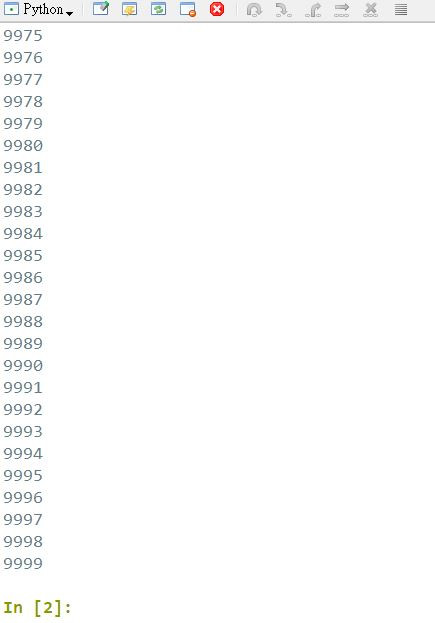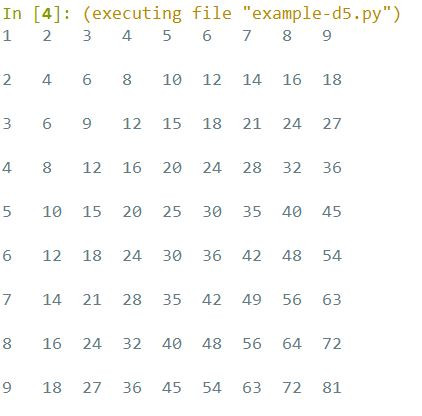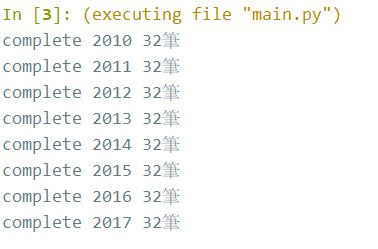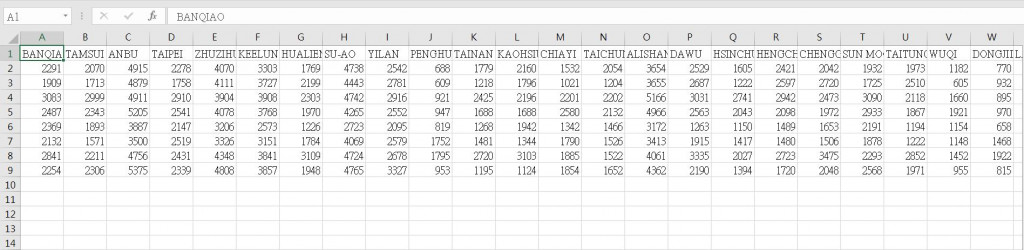#DAY 5
0

## Day5-上萬筆資料我該怎麼辦

``````for i in range(10000):
print(i)
``````## For 迴圈

#### 練習5-1

``````for i in range(1,10):
for j in range(1,10):
print(i*j,end="\t") #"\t" 相當於 tab
print("\n") #"\n" 是 Windows 內的換行符
``````

Output:## While 迴圈

``````#無限迴圈範例
while(1 == 1):
print("In while loop...")
``````

``````key = "1"
while(key != "0"):
key = input("Enter a letter:")
print("You enter " + key)
print("Exit")
``````

## 應用時機

``````"""
2014年無屏東資料
2013年前無新屋測站資料
"""
import json
import csv

year_list = ["2010","2011","2012","2013","2014","2015","2016","2017"]
rainFall_yearlist = []
location_list = ['BANQIAO', 'TAMSUI', 'ANBU', 'TAIPEI', 'ZHUZIHU', 'KEELUNG', 'HUALIEN', 'SU-AO', 'YILAN', 'PENGHU', 'TAINAN', 'KAOHSIUNG', 'CHIAYI', 'TAICHUNG', 'ALISHAN', 'DAWU', 'HSINCHU', 'HENGCHUN', 'CHENGGONG', 'SUN MOON LAKE', 'TAITUNG', 'WUQI', 'DONGJIDAO', 'LANYU', 'KINMEN', 'MATSU', 'PENGJIAYU', 'YUSHAN', 'TAOYUAN', 'CHANGHUA', 'MIAOLI', 'YUNLIN']

filepath = r"C:\\User\\python\\data\\"

for year in year_list:

file = open(filepath + year + ".json", 'r', encoding='UTF-8')
file.close()

rainFall_list = []

index = 0
for item in data["dataset"]["location"]:
rainFall_total = 0.0

if(item["locationName"] != "PINGTUNG,屏東" and item["locationName"] != "XINWU,新屋"):
index += 1
for elem in item["weatherElement"]["time"]:
it = elem["elementValue"]["value"]
if(str(it) != "T" and str(it) != "X"):
if(float(it) > 0.0):
rainFall_total += float(it)

rainFall_list.append(int(rainFall_total)) #數字優化

rainFall_yearlist.append(rainFall_list)
print("complete " + year + " " + str(index) + "筆")

with open(filepath + r"csv\\output.csv', 'w', newline='') as csvfile:
writer = csv.writer(csvfile)

writer.writerow(location_list)

for item in rainFall_yearlist:
writer.writerow(item)
``````csv 檔案輸出：